Practice Test: Civil Engineering (CE)- 11

# Practice Test: Civil Engineering (CE)- 11

Test Description

## 65 Questions MCQ Test GATE Civil Engineering (CE) 2023 Mock Test Series | Practice Test: Civil Engineering (CE)- 11

Practice Test: Civil Engineering (CE)- 11 for Civil Engineering (CE) 2023 is part of GATE Civil Engineering (CE) 2023 Mock Test Series preparation. The Practice Test: Civil Engineering (CE)- 11 questions and answers have been prepared according to the Civil Engineering (CE) exam syllabus.The Practice Test: Civil Engineering (CE)- 11 MCQs are made for Civil Engineering (CE) 2023 Exam. Find important definitions, questions, notes, meanings, examples, exercises, MCQs and online tests for Practice Test: Civil Engineering (CE)- 11 below.
Solutions of Practice Test: Civil Engineering (CE)- 11 questions in English are available as part of our GATE Civil Engineering (CE) 2023 Mock Test Series for Civil Engineering (CE) & Practice Test: Civil Engineering (CE)- 11 solutions in Hindi for GATE Civil Engineering (CE) 2023 Mock Test Series course. Download more important topics, notes, lectures and mock test series for Civil Engineering (CE) Exam by signing up for free. Attempt Practice Test: Civil Engineering (CE)- 11 | 65 questions in 180 minutes | Mock test for Civil Engineering (CE) preparation | Free important questions MCQ to study GATE Civil Engineering (CE) 2023 Mock Test Series for Civil Engineering (CE) Exam | Download free PDF with solutions
 1 Crore+ students have signed up on EduRev. Have you?
Practice Test: Civil Engineering (CE)- 11 - Question 1

### Which of the following curves represents the Y= In (|e| (sin sin (|x|)|) for |x| < 2π?

Detailed Solution for Practice Test: Civil Engineering (CE)- 11 - Question 1 Y = In (|(e|(sin sin(|x||)|)

= In (|(e| (sin sin(|x|)|)=|sin sin(|x|)|

Now for drawing the graph follow these steps

1) Draw sinx graph on paper

2) Take reflection about x-axis, you will get graph of sin(|x|)

3) Now, take a reflection again but this time

about y-axis, you will get the graph of sin(|x|)

⇒ Option c is correct.

Practice Test: Civil Engineering (CE)- 11 - Question 2

### Direction: In the following question, a sentence is given with blanks to be filled in with appropriate word(s). Four alternatives are suggested for the question. Choose the correct alternative out of the four.The _______ of the moment led Lin to post on Craigslist, describing the guy's _____ shirt and partially dyed blond hair.

Detailed Solution for Practice Test: Civil Engineering (CE)- 11 - Question 2 Option A: Serendipity is the occurrence and development of events by chance in a happy or beneficial way. ‘Plaid shirt’ is made up of flannel and worn during the winter.

Option B: Profanity is socially offensive language, which may also be called bad or offensive language. ‘Profanity of a moment’ makes no sense. Therefore, this option can’t be used.

Option C: Penchant is a strong or habitual liking for something or tendency to do something. ‘Penchant of a moment’ makes no sense. Therefore, this option can’t be used.

Option D: Predilection is a preference or special liking for something; a bias in favour of something. ‘Slurry’ is a thin sloppy mud or cement or, in extended use, any fluid mixture of a pulverized solid with a liquid usually water, often used as a convenient way of handling solids in bulk. Thus, this option makes no sense in the context of the sentence.

Therefore, option a is the apt answer.

Analogy = Situation: Appearance. None of the options accept A showcase any situation. Hence A is the right one. The word moment is present in the question, hence any word which suits situation would be the right option.

Practice Test: Civil Engineering (CE)- 11 - Question 3

### A number 18567332145x is divisible by 8. What can be the minimum value of x?

Detailed Solution for Practice Test: Civil Engineering (CE)- 11 - Question 3 For a number to be divisible by 8; its last 3 digits should be divisible by 8

So 45x is divisible by 8

The number is 45 which is near to 48, the number which gets divided by 6 (8 × 6 = 48)

Thus x = 6 is the correct answer

Practice Test: Civil Engineering (CE)- 11 - Question 4

The simple interest on a certain sum for 2 years at 9% per annum is Rs. 225 less than the compound interest on the same sum for 2 years at 10% per annum. The sum is:

Detailed Solution for Practice Test: Civil Engineering (CE)- 11 - Question 4 % Simple interest on sum for 2 year = 2×9 = 18%

% compound interest for 2 year = 10+10+(10×10)/100 = 21%

difference in interest = 225 Rs.

So (21% - 18%) = 3% of sum = 225 Rs.

So Sum = 225×100/3 = 7500 Rs.

Practice Test: Civil Engineering (CE)- 11 - Question 5

Which of the following is the MOST OPPOSITE in meaning to Obstreperous?

Detailed Solution for Practice Test: Civil Engineering (CE)- 11 - Question 5 Obstreperous= noisy and difficult to control

Sophisticated= Cultured or one who speaks in a respectable manner

Blusterous = loud and aggressive.

Ribald = indecent or bawdy.

Imperative= essential

Practice Test: Civil Engineering (CE)- 11 - Question 6

Consider a function f(x)=1-|x| on -1≤x≤1.The value of x at which the function attains a maximum, and the maximum value of function are:((mathematics:maxima,minima)

Detailed Solution for Practice Test: Civil Engineering (CE)- 11 - Question 6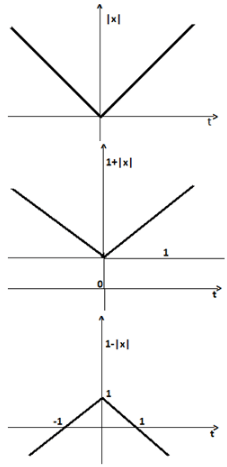Practice Test: Civil Engineering (CE)- 11 - Question 7

An article is sold with a certain profit percentage such that selling the same at one-third price, there will be a loss of 60%. Find the certain profit percentage.

Detailed Solution for Practice Test: Civil Engineering (CE)- 11 - Question 7

Let the selling price of the article =Rs.x

If the article is sold in 1/3 price, then selling price =Rs.x/3 and loss =60%

So, the cost price of the article = Rs.( x/3 ) × (100/40) = Rs. (5x/6) Then, profit earned by selling the article at Rs. x = Rs. x – (5x/6) = Rs.x/6

∴ The required profit percentage = [(x/6)/ (5x/6) × 100]% = 20%.

Alternatively:-

Say CP = 100 Rs. say profit X%

SP = 100 + X

New SP = (100 + X)/3 = 100 - 60

X = 20

Alternative Method: (Easier Method)

Let cost price =100 (Because percentages are mentioned here, and the highest number will be 100)

Loss = 60%

Selling price = 40

Actual selling price = 3 × 40 = 120

Profit = 20 %

Practice Test: Civil Engineering (CE)- 11 - Question 8

Rs. 200 are divided among Arun, Bholu and Chetan such that Arun's share is Rs. 30 more than Bholu's and Rs. 20 less than Chetan's. What is Bholu's share?

Detailed Solution for Practice Test: Civil Engineering (CE)- 11 - Question 8

It is given that Rs. 200 divided among Arun, Bholu and Chetan

Let Bholu’s share = X Rs.

Arun’s Share = X +30 Rs

Chetan’s Share = X+30+20 = X+50

Now, Arun+ Bholu+ Chetan = (X+30) +(X) +(X+50) = 200

3X = 200 – 80

X = 120/3

X = 40

Bholu’s share is 40 Rs.

Hence, (b) is correct option.

Practice Test: Civil Engineering (CE)- 11 - Question 9

Average marks in a test conducted in a class of 24 students gave the average score as 56. It was detected that while computing the average, marks of 3 students were taken as 46, 47 and 43 instead of 64, 74 and 34 respectively. Which of the following is the most appropriate description of the percentage by which the class average goes up now?

Detailed Solution for Practice Test: Civil Engineering (CE)- 11 - Question 9

The increase in total marks of the class is (64–46)+(74–47)+(34–43) = 36

The average goes up by 36/24 = 1.5 marks

Increase in %age terms = 1.5 x 100/56 = 2.67%.

So, option d is correct.

Practice Test: Civil Engineering (CE)- 11 - Question 10

Present average age of Kanika and Shweta is ‘x’ years. Ratio of the age of Kanika one year ago to the age of Monika four year hence will be 1: 2. Present average age of Kanika, Monika and Shweta is 22 years. Find the value of ‘x’ if the present age of Kanika is 16 years.

Detailed Solution for Practice Test: Civil Engineering (CE)- 11 - Question 10

Present age of Kanika = 16 years

Age of Kanika one year ago = 16 – 1 = 15 years

Age of Monika after four years = 15 × 2 = 30 years

Present age of Monika = 30 – 4 = 26 years

Sum of the present ages of Kanika, Monika and Shweta = 22 × 3 = 66 years

Present age of Shweta = 66 – 16 – 26 = 24 years

Present average age of Kanika and Shweta = x = (24+16)/2=20 years

So, the value of ‘x’ is 20 years

So option (d) is the correct answer.

Practice Test: Civil Engineering (CE)- 11 - Question 11

The dip of the compass needle ______.

Detailed Solution for Practice Test: Civil Engineering (CE)- 11 - Question 11 The angle that a magnetic needle makes with the horizontal plane at any specific location. Magnetic dip is 0 ° at the magnetic equator and 90 ° at each of the magnetic poles. Also called magnetic inclination.
Practice Test: Civil Engineering (CE)- 11 - Question 12

The Sludge Volume Index for mixed liquor having suspended solids concentration of 2000 mg/l and showing a settled volume of 200 ml from a one-litre sample would be

Detailed Solution for Practice Test: Civil Engineering (CE)- 11 - Question 12 The sludge volume index (SVI) is the volume in millilitres occupied by 1 g of a suspension after 30 min settling.

S.V.I = 200 ml/2000 mg

= 200 ml/2 gm = 100 ml/gm

Practice Test: Civil Engineering (CE)- 11 - Question 13

The velocity field for flow is given by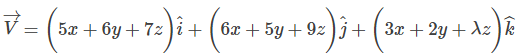and the density varies as p = po exp (-2t). In order that the mass is conserved, the value of λ should be;

Detailed Solution for Practice Test: Civil Engineering (CE)- 11 - Question 13 From 3D continuity equation for compressible flow,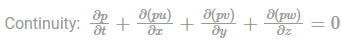By putting the given values in the above equation,

ρoe-2t(-2) + ρ.5+ ρ.5 + ρ. λ = 0

Since ρ = ρo e-2t

Hence

-2ρ + ρ.5+ ρ.5+ ρ. λ = 0

-2+5+5+ λ = 0

Hence λ = -8

Practice Test: Civil Engineering (CE)- 11 - Question 14

In the influence line diagram for mid-span bending moment of a simply supported beam, the ordinate at the quarter span is 0.5 m. If the span of the beam is doubled, the ordinate at the mid-span of the influence line will be;

Detailed Solution for Practice Test: Civil Engineering (CE)- 11 - Question 14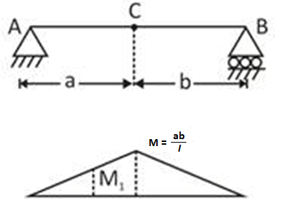JLD of bending moment at C

For the above problem [a = b = 1/2]

M = ab/l = 1/4

Since length of the span is doubled

l' = 2l

Hence the ordinate of M1 will now be = 0.5 × 2 = 1 m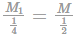M = 2M1

∴ Ordinate of M at mid span = 2 × 1 = 2m

Practice Test: Civil Engineering (CE)- 11 - Question 15

The incorrect statements is/are;

Detailed Solution for Practice Test: Civil Engineering (CE)- 11 - Question 15
• Ca(OH)2 is not a desirable product in the concrete mass because it is soluble in water and gets leached easily, particularly in hydraulic structure, that’s why cement with the small percentage of C3S and more C2S is recommended for use in the hydraulic structure.

• The concrete continues to harden over several months. Hardening is not a drying process and can very well take place in water. Heat speeds up the setting and hardening of cement

• The stiffening of cement without strength development (flash setting of cement) may cause because of C3A or C4AF.

So option B statement is incorrect.

Practice Test: Civil Engineering (CE)- 11 - Question 16

A portal frame shown in figure (not drawn to scale) has a hinge support at joint P and a roller support at joint R. A point load of 50 kN is acting at joint R in the horizontal direction. The flexural rigidity. EI, of each member is 106 kNm2. Under the applied load, the horizontal displacement (in mm, round off to 1 decimal place) of joint R would be __________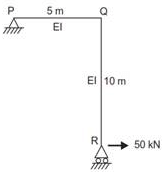Detailed Solution for Practice Test: Civil Engineering (CE)- 11 - Question 16 For reaction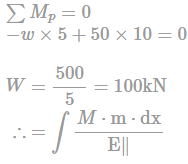When unit load at R is acting in the direction of 50kN load, then reaction at R = 2 (downward)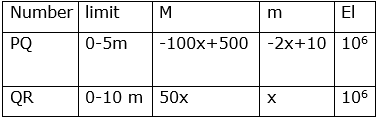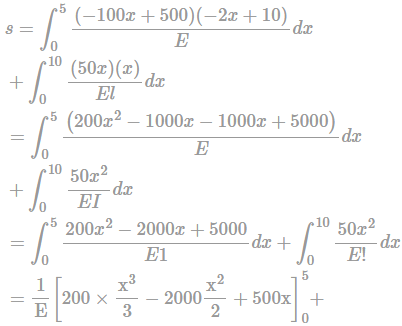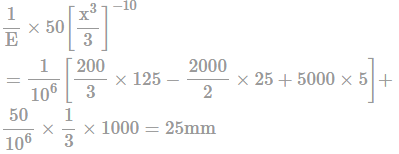Alternatively: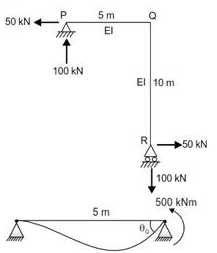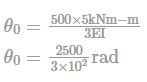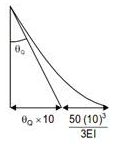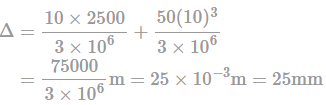Practice Test: Civil Engineering (CE)- 11 - Question 17

A plane frame is as shown in the figure below. If MBA = 10 kNM (clockwise) and MCD = 21 kNM (counter clockwise) then the value of load P is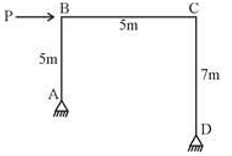Detailed Solution for Practice Test: Civil Engineering (CE)- 11 - Question 17 For body diagram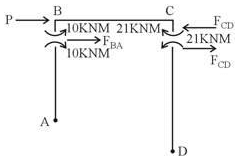Applying ΣMA = 0

–FBA × 5 + 10 = 0

FBA = 2 kN

Similarly, ΣMD = 0

FCD × 7 – 4 = 0

FCD = 3 kN

For horizontal equilibrium of BC

P + FBA – FCD = 0

P = 1 kN

Practice Test: Civil Engineering (CE)- 11 - Question 18

A circular tank of base diameter 12m is subjected to a total load of 12,000 kN. Calculate the vertical stress (in kN/m2) at a point P which is at a depth of 3m and 2m away from the centre of the loaded area.

Detailed Solution for Practice Test: Civil Engineering (CE)- 11 - Question 18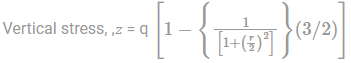Given load, Q = 12000 kN

Depth, z = 3m

Area of tank, A = πr2 = 113.04 m2

q = 12000/113.04 = 106.16 kN/m2

σz =18.05 kN/m2
Practice Test: Civil Engineering (CE)- 11 - Question 19

The results of two plate load tests performed on a given location with two circular plates are given below:

1. Diameter = 750 mm, S = 15 mm, Q = 150 kN

2. Diameter = 300 mm, S = 15 mm, Q = 50 kN

Use Housel’s equation i.e. Q = Aq + Ps

A = contact area

q = bearing pressure beneath area A (constant)

P = perimeter of footing

s = Perimeter shear (constant)

Determine the load (in kN) on circular footing 1.2 m diameter that will cause a settlement of 15 mm.

Detailed Solution for Practice Test: Civil Engineering (CE)- 11 - Question 19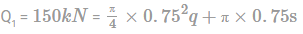⇒ 150 = 0.44q + 2.36s …(i)

Similarly

Q2 = 50 kN

⇒ 50 = 0.071q + 0.94s ...(ii)

Solving (i) and (ii)

S = 46.13 kN/m

q = 93.48 kN/m2

Now for footing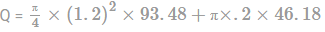Q = 279.6 kN

Practice Test: Civil Engineering (CE)- 11 - Question 20

The net safe bearing capacity of a purely cohesive soil

Detailed Solution for Practice Test: Civil Engineering (CE)- 11 - Question 20 For a cohesive soil ø = 0 hence, Nc = 5.7, Nq = 1 and Nγ = 0

Thus according to Terzaghi’s equation the net safe bearing capacity will be independent of both width and depth of the footing.

Practice Test: Civil Engineering (CE)- 11 - Question 21

A scale representing either three units or only one unit and its fractions up to second place of decimal point is _______.

Detailed Solution for Practice Test: Civil Engineering (CE)- 11 - Question 21 A scale representing either three units or only one unit and its fractions up to second place of decimal point is known as the diagonal scale, while plane scale is represents only two dimension.
Practice Test: Civil Engineering (CE)- 11 - Question 22

If f(t) = (eat - cos bt) 1/t , then the Laplace transform of f(t)

Detailed Solution for Practice Test: Civil Engineering (CE)- 11 - Question 22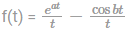We know that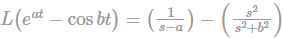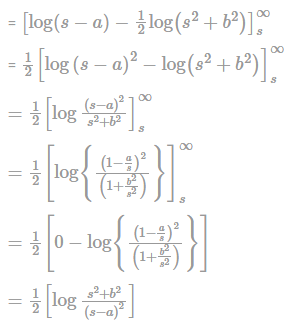Practice Test: Civil Engineering (CE)- 11 - Question 23

A concrete beam pre-stressed with a parabolic tendon is shown in the fig. The pre-stressing force applied is 1620 kN. The ULD includes self-weight of the beam. The stress in the top fibre of the middle section is (take compressive stress as positive)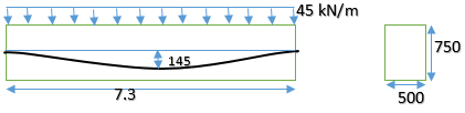Detailed Solution for Practice Test: Civil Engineering (CE)- 11 - Question 23 Area , A = 500 × 750 = 375,000 mm2

Bending Moment at min-span, M = (45 × 7.32)/8

= 299.7 kNm

Stress At top fibre fct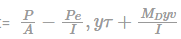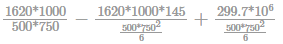= 5.7 N/mm2

Practice Test: Civil Engineering (CE)- 11 - Question 24

Consider a signal x (t) given by x(t) L.T. ↔ x(s) = log(s+5/s+6) , then x(t) is given by

Detailed Solution for Practice Test: Civil Engineering (CE)- 11 - Question 24 If L[f(t)] = F[S]

Then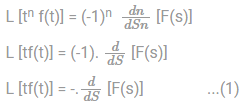Given F(S) = x(S) = log log (S+5) -log log (5+6)

Differentiate both sides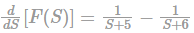Multiply by negative sign on both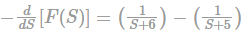By property (1)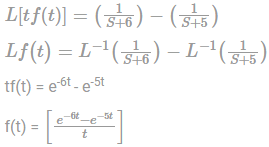Practice Test: Civil Engineering (CE)- 11 - Question 25

Consider the following simultaneous equations (with c1, and c2 being constants):

3x1 + 2x2 = c1

4x1 + x2 = c2

The characteristic equation for these simultaneous equations is;

Detailed Solution for Practice Test: Civil Engineering (CE)- 11 - Question 25 given systems

3x1 + 2x2 = c1

4x1 + x2 = c2 Matrix From is (3 2 4 1 )(x1 x2) = [c1 c2]

AX = B

Characteristic equations of above systems is

|A - λI| = 0

|3 - λ 2 4 1-λ | = 0

By expanding λ2 - 4λ - 5 = 0

Practice Test: Civil Engineering (CE)- 11 - Question 26

The degree of static indeterminacy of the truss shown below is.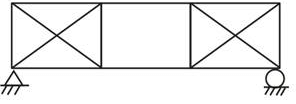Detailed Solution for Practice Test: Civil Engineering (CE)- 11 - Question 26 Ds = m + r - 2j

= 14 + 3 - 2 × 8

= 17 - 16

= 1

*Answer can only contain numeric values
Practice Test: Civil Engineering (CE)- 11 - Question 27

A pressure gauge reads 62.3 kPa and 90 kPa respectively at heights of 7 m and 4 m fitted on the side of a tank filled with liquid. What is the approximate density of the liquid on kg/m3?

Detailed Solution for Practice Test: Civil Engineering (CE)- 11 - Question 27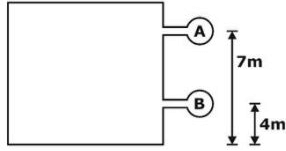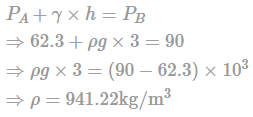Practice Test: Civil Engineering (CE)- 11 - Question 28

Which one of the statement is INCORRECT in design of hourly traffic volume?

Detailed Solution for Practice Test: Civil Engineering (CE)- 11 - Question 28 30th highest hourly traffic volume is the hourly volume that will be exceeded only 29 times in a year and all other hourly volumes of the year will be less than this value.
Practice Test: Civil Engineering (CE)- 11 - Question 29

A lift irrigation scheme using a discharge of 81m3/hr is planned to raise a crop whose delta is 45cm. Intensity of irrigation is 55%. Assuming 4500 hours of working of water supply for a year, area(hectares) required for irrigation would be;

Detailed Solution for Practice Test: Civil Engineering (CE)- 11 - Question 29 Total volume of water applied in a year = 81 x 4500 = 364500m3

Now the area to be irrigated = 364500/0.45 = 810000 = 81hectare

Required area = 81 /0.55 = 147.2 hectares

Practice Test: Civil Engineering (CE)- 11 - Question 30

An 8 m long simply-supported elastic beam of rectangular cross-section 100mm × 200 mm is subjected to a uniformly distributed load of 10 kN/m over its entire span. The maximum principal stress (in MPa, up to two decimal places) at a point located at the extreme compression edge of a cross-section and at 2 m from the support is ____________.

Detailed Solution for Practice Test: Civil Engineering (CE)- 11 - Question 30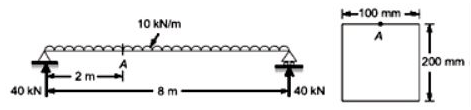MA = (−10 × 2 × 1) + 40 × 2 = 60 k Nm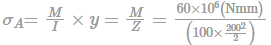= 90/mm3

τ = 0N/mm2 {point is at top}

So principal stress = 90N/mm2 = 90MPa

Practice Test: Civil Engineering (CE)- 11 - Question 31

The anchorage value of a standard bend, of a reinforcement bar of diameter φ

Detailed Solution for Practice Test: Civil Engineering (CE)- 11 - Question 31 Standard bend = 90° bend

Anchorage value for 90° bend = 8φ

Practice Test: Civil Engineering (CE)- 11 - Question 32

What is the anchorage length of a tie bar, which is bent through 135° round a bar of diameter 12mm?

Detailed Solution for Practice Test: Civil Engineering (CE)- 11 - Question 32 For secondary reinforcement such as stirrups in beams and transverse ties in a column, complete development length and anchorage shall be deemed to have been provided when the bar is bent through an angle of 135° and is continued beyond the end of the curve for a length of at least 6 diameters.

So, 6φ = 6 x 12 = 72 mm

Practice Test: Civil Engineering (CE)- 11 - Question 33

If two centrifugal pumps (I and II) with heads H1, HII and discharge Q1, QII are connected in series, then the correct option is:

Detailed Solution for Practice Test: Civil Engineering (CE)- 11 - Question 33 When two centrifugal pumps (I and II) are joined in series with head (HI + II) results as sum of the individual pumps heads where flow rate remains the same, so HI + II = H1 + HII, QI+II = QI = QII.
Practice Test: Civil Engineering (CE)- 11 - Question 34

A laboratory model of a river is built to a geometric scale of 1:200. The fluid used in the model is oil of mass density 850 kg/m3. The highest flood in the river is 12000 m3/s. the corresponding discharge in the model shall be

Detailed Solution for Practice Test: Civil Engineering (CE)- 11 - Question 34 Scale ratio, Lr = 1/200

Flow in river is based on gravitational force. So, model will be based on Froude number.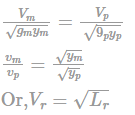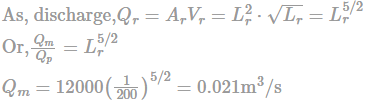Practice Test: Civil Engineering (CE)- 11 - Question 35

A stone weights 450 N in air and 250 N in water. The volume of the stone is:

Detailed Solution for Practice Test: Civil Engineering (CE)- 11 - Question 35 Weight of stone in water = weight of stone in air – (Ywater × vol of stone)

250 = 450 - 9810 × V

V = 0.0204 m3

*Answer can only contain numeric values
Practice Test: Civil Engineering (CE)- 11 - Question 36

A beam is built using M25 concrete and Fe-250 bars of 16 mm dia bars. In limit state design, the development length, Ld, in terms of diameter of reinforcement, for compression zone is _______ mm.

[τbd = 1.4 N/mm2]

Detailed Solution for Practice Test: Civil Engineering (CE)- 11 - Question 36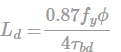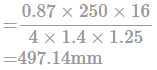Factor 1.25 is used for compression.

Practice Test: Civil Engineering (CE)- 11 - Question 37

The system of equations x - 4y + 7z = 12 , 3x + 8y - 2z = 10, 26 z - 8y = 6

Detailed Solution for Practice Test: Civil Engineering (CE)- 11 - Question 37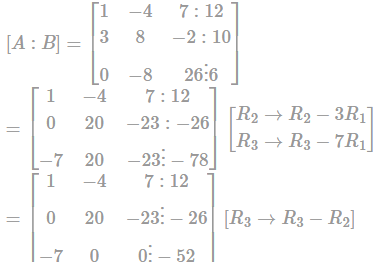Here, Rank [A : B] = Rank⁡[A] = 3 = n

And n = 3

Therefore, the system has unique solution.

*Answer can only contain numeric values
Practice Test: Civil Engineering (CE)- 11 - Question 38

A steel bar of 20 mm Fe415 grade is embedded in a concrete block of M20 grade. The bond stress τbd = 1.2 MPa as per IS : 456-2000, for mild steel in tension. The length of embedment is 1m. The maximum pulling force that can be applied on the bar is ___________ kN

Detailed Solution for Practice Test: Civil Engineering (CE)- 11 - Question 38 Force required to pull out the bar from concrete

P1 = τbd × 1.6 × π × d × l = 1.2 × 1.6 × π × 20 × 1000 × 10-3

P1 = 120.63 kN

Force required to break the bar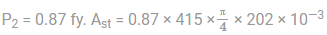P2 = 113.43 kN

Hence maximum pulling force = Min. of P1 & P2

= 113.43 kN

Practice Test: Civil Engineering (CE)- 11 - Question 39

A single-stage impulse turbine with a diameter of 120 cm runs at 3000 rpm. If the blade speed ratio is 0.42, then, the inlet velocity of steam will be

Detailed Solution for Practice Test: Civil Engineering (CE)- 11 - Question 39

D = 120 cm = 0.120 m , N = 3000 rpm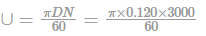= 60π

Actual Inlet Velocity

= 60π/0.42 = 448.79m/s

Practice Test: Civil Engineering (CE)- 11 - Question 40

A penstock is 3000 meters long. Pressure wave travels in it with a velocity of 1500 m/s. If the turbine gates are closed uniformly and completely in a period of 4.5 seconds, then it is called

Detailed Solution for Practice Test: Civil Engineering (CE)- 11 - Question 40

L = 3000 m

C = 1500 m/s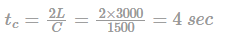t = 4.5 sec > tc hence slow closure

Practice Test: Civil Engineering (CE)- 11 - Question 41

Choose the correct statement(s) with respect to the Lag distance in computing the absolute minimum sight distance of the vehicle.

i. The reaction time is taken as 0.15sec

ii. The distance is dependent only on the coefficient of friction, reaction time and gradient

iii. It is depended on reaction time and velocity.

iv. The reaction time is the time required to observe the obstruction.

Detailed Solution for Practice Test: Civil Engineering (CE)- 11 - Question 41

The lag distance is given by the simple equation.

Lag distance = Velocity x Reaction Time

Reaction time as per IRC recommendation is 2.5 sec

Lag distance is dependent mainly on Velocity of the moving vehicle and the reaction time and independent on coefficient of longitudinal friction, gradient and efficient of brake.

The reaction time of the driver is the time required to apply the brake after observing the obstruction

Practice Test: Civil Engineering (CE)- 11 - Question 42

Considering two vehicles moving at a speed of 85kmph and 60 kmph on a road surface with skid resistance of 0.72 and braking efficiency of 55%, what will be the non-passing sight distance? Assume necessary data.

Detailed Solution for Practice Test: Civil Engineering (CE)- 11 - Question 42

Tag v1 = 85 kmph

V2 = 60 kmph

Skid resistance = 0.72

Brake efficiency, ŋ = 55%

Coefficient of friction, f = Skid resistance x Brake efficiency

f = 0.72 x 55/100

f = 0.396 = 0.40

Non- passing sight distance = SSD1 + SSD2

Assume reaction time , t = 2.50 secc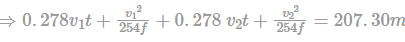*Answer can only contain numeric values
Practice Test: Civil Engineering (CE)- 11 - Question 43

length of runway under standard condition is 1800m. the airport site has an elevation of 300m. it has reference temperature of 33°C. if the runway is to be constructed with effective gradient of 0.6%. then determine the corrected runway length

Detailed Solution for Practice Test: Civil Engineering (CE)- 11 - Question 43 i) Correction for elevation = 7/100 × 1800 × 300/300 = 126 m

Corrected length = 1926 m

ii) Standard atmospheric temp of the given elevation

= 15° = 0.0065 × 300 = 13.05° C

Rise in temp = 33°C – 13.05° C = 19.95° C

Correction = 1926 / 100 × 19.95 = 384.237 m

Corrected length = 1926 + 384.237 = 2310.23 m

Check for combine correction = Total correction in %

= 2310.23 – 1800 / 1800 = 28.34%

28.34% < 35%

Hence, OK

Correction for gradient = 20/100 × 2310.23 × 0.6 = 277.22 m

Corrected length = 2310.23 + 277.22 = 2587.45 m

Practice Test: Civil Engineering (CE)- 11 - Question 44

A vehicle moving at 40 kmph speed was stopped by applying the brake and the length of skidmark was 18 m. If the average skid resistance of the pavement is known to be 0.70, the brake efficiency of the the test vehicle (in %) is

Detailed Solution for Practice Test: Civil Engineering (CE)- 11 - Question 44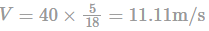Average skid resistance developed,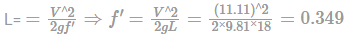∴ Brake efficiency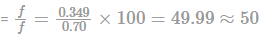Practice Test: Civil Engineering (CE)- 11 - Question 45

A rectangular cross-section as shown below, experiences a maximum bending stress of 30 N/mm2. The magnitude of force experienced by the shaded region is _____ kN.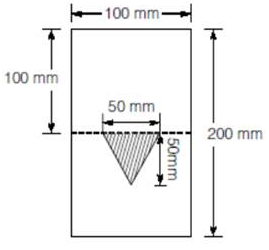Detailed Solution for Practice Test: Civil Engineering (CE)- 11 - Question 45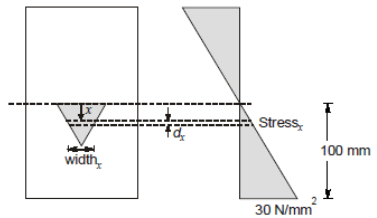Force experienced by infinity small area at distance x = dF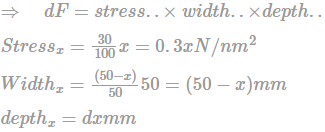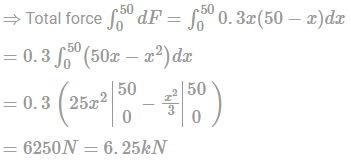Practice Test: Civil Engineering (CE)- 11 - Question 46

Flexibility matrix of the beam shown below is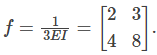If the support B settles by Δ/EI units, what is the reaction at B?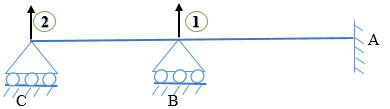Detailed Solution for Practice Test: Civil Engineering (CE)- 11 - Question 46

From relation Δ = f.P

In matrix form

Δ1 = f11P1 + f12P2 .................i

Δ2 = f21P1 + f22P2...............ii

Using given data,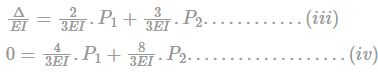Solving eqn (iii) and (iv)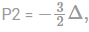∴ Reaction at B P1 = 3.75 Δ

Practice Test: Civil Engineering (CE)- 11 - Question 47

The stiffness matrix of a beam element is given as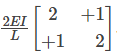. Then the flexibility matrix is

Detailed Solution for Practice Test: Civil Engineering (CE)- 11 - Question 47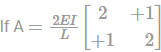is stiffness matrix, then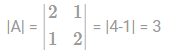The matrix of co-factor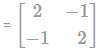Transpose matrix,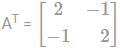Inverse of Matrix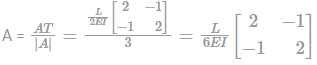Practice Test: Civil Engineering (CE)- 11 - Question 48

What are the critical activities in the project network shown below. (Use PERT analysis)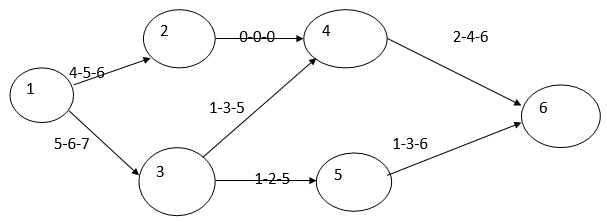Detailed Solution for Practice Test: Civil Engineering (CE)- 11 - Question 48

First find the expected time of the activities. Then found slack of the activities. Activities having zero slack are the critical activities.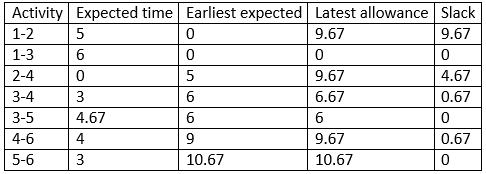The critical activity is 1-3-5-6.

*Answer can only contain numeric values
Practice Test: Civil Engineering (CE)- 11 - Question 49

A rectangular concrete beam of width 150 mm and depth 250 mm is prestressed by pretensioning to a force of 200 kN at an eccentricity of 30 mm. the cross-sectional area of the prestressing steel is 200 mm2. Take modulus of elasticity of steel and concrete as 2.1 x 105 MPa and 3 x 104 MPa respectively. The percentage loss of stress in the prestressing steel due to elastic deformation of concrete is ________.

Detailed Solution for Practice Test: Civil Engineering (CE)- 11 - Question 49 Stress in concrete,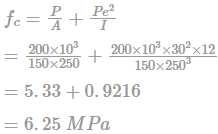Loss of prestress due to elastic deformation,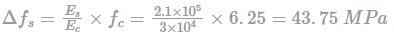Total stress in steel,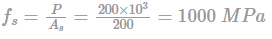Percentage loss of stress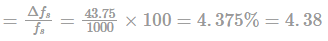Practice Test: Civil Engineering (CE)- 11 - Question 50

A concrete beam prestressed with a parabolic tendon is shown in the sketch. The eccentricity of the tendon is measured from the centroid of the cross-section. The applied prestressing force at service is 1620KN. The uniformly distributed load of 45 KN/m includes self-weight.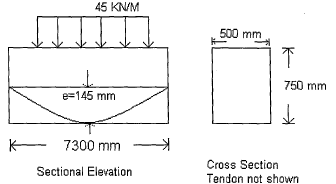The stress (in N/mm2) in the bottom fibre at Mid Span is

Detailed Solution for Practice Test: Civil Engineering (CE)- 11 - Question 50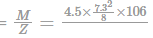= -6.3948N/mm2

Stress due to pre-stres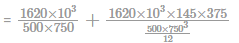= 9.3312N/mm2

Total stress = 9.3312 – 6.3948 = 2.9364 N/mm2 = 2.94 Compression

Practice Test: Civil Engineering (CE)- 11 - Question 51

A thick cylinder of internal diameter 10 cm is subjected to an internal pressure of 50 N/mm2, then the thickness of the cylinder according to the maximum shear stress theory, if yield stress is 270 N/mm2 and factor of safety is 2, is _______mm

Detailed Solution for Practice Test: Civil Engineering (CE)- 11 - Question 51

Inner radius, R1 = 50 mm

Let external radius is R2 mm

Radial pressure, p = 50 N/mm2

Principal stresses at inner surface of thick cylinder are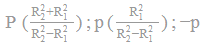Maximum shear stress, τmax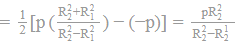Allowable normal stress, σa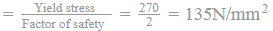Allowable shear stress.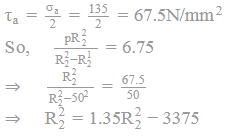Undefined control sequence \therefore

Thickness of cylinder,

t = R2 − R1 = 98.2 − 50 = 48.2mm

Practice Test: Civil Engineering (CE)- 11 - Question 52

Calculate the standard error of the volume of a cuboid whose sides are A, B, and C have values given below and standard error as +/-___________ in cm3

A = 50.0 cm +/-.04 cm

B = 40.0 cm +/-.03 cm

C = 30.0 cm + /-.02 cm

Detailed Solution for Practice Test: Civil Engineering (CE)- 11 - Question 52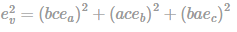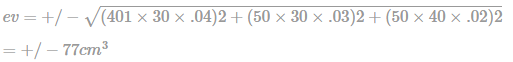Practice Test: Civil Engineering (CE)- 11 - Question 53

A water mains 140 cm inner diameter contains water at a pressure head of 200 m. The minimum thickness of the metal shell required for the water mains, given that maximum permissible stress in the metal is 400 kg/cm2, will be ___ mm.

Detailed Solution for Practice Test: Civil Engineering (CE)- 11 - Question 53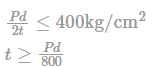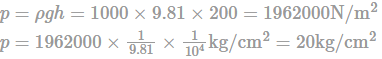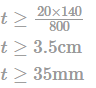Practice Test: Civil Engineering (CE)- 11 - Question 54

FEM for the given beam is: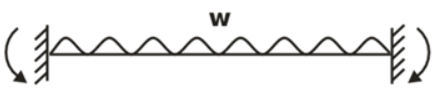Detailed Solution for Practice Test: Civil Engineering (CE)- 11 - Question 54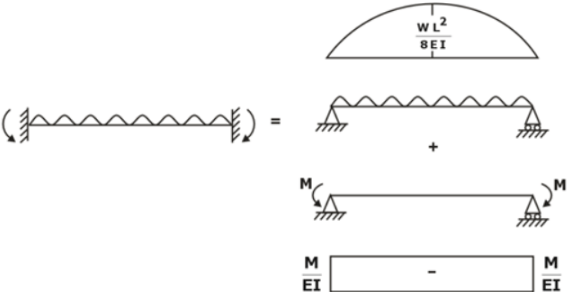Compatibility equation θA = 0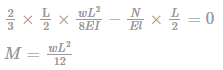Hence, the correct option is (A).

*Answer can only contain numeric values
Practice Test: Civil Engineering (CE)- 11 - Question 55

A 50 cm diameter pipe carries water (v = 0.294 × 10–6 m2/s) at an average velocity of 10 m/s . The roughness of pipe surface is 0.8 mm. For fully rough turbulent flow, What is the boundary shear stress.

Detailed Solution for Practice Test: Civil Engineering (CE)- 11 - Question 55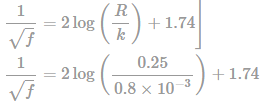F = 0.022

Shear velocity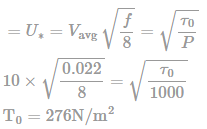Practice Test: Civil Engineering (CE)- 11 - Question 56

Determine the distance from the pipe wall at which local velocity is equal to the average velocity for turbulent flow in pipe

Detailed Solution for Practice Test: Civil Engineering (CE)- 11 - Question 56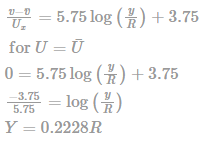Practice Test: Civil Engineering (CE)- 11 - Question 57

A water supply system is designed for a town with population of 2,50,000. Determine the fire demand (in ml/min) using empirical relationship and the Match List I and List II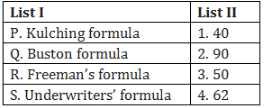Detailed Solution for Practice Test: Civil Engineering (CE)- 11 - Question 57

Kulching Formula

Q = 3182 √P

P = population in thousand; Q = demand in lit/min

Q = 50311.8 lit/min

Freeman Formula:

Q = 1136[(P/10 )+ 10]

Q = 39760 lit/min

USA- Underwriters formula

Q = 4637 √P [1- 0.01√P ]

Q = 61725 lit/min

Buston Formula:

Q = 5663 √P = 89540 lit/min

Practice Test: Civil Engineering (CE)- 11 - Question 58

Determine the critical deficit (in ppm) of dissolved oxygen when the stream flowing at a rate of 210 cumec with saturated dissolved oxygen limit of 10.6ppm is diluted with a sewage flowing at the rate of 1.35 cumec. Assume the Dissolved oxygen limit of waste water as 4ppm.

Detailed Solution for Practice Test: Civil Engineering (CE)- 11 - Question 58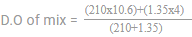D.O. of mix = 10.55 ppm

Critical deficit of D.O. = Saturated D.O. of stream – D.O. of mix = 0.05 ppm

Practice Test: Civil Engineering (CE)- 11 - Question 59

Which of the following are correctly matched:

1. Turbulent flow = Moment Integral equation

2. Laminar flow = Hagen Poiseuille equation

Detailed Solution for Practice Test: Civil Engineering (CE)- 11 - Question 59 Hagen poiseuille equation considers the Laminar flow in a pipe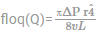P = Pressure difference across tube

Μ = viscosity of liquid.

In turbulent flow, prantis mixing length theory is used to calculate shear stress.

Practice Test: Civil Engineering (CE)- 11 - Question 60

12m3/s of water is applied to a field having area of 30 hectares for 4 hours. About 0.3m of water stored in the root zone. Water application efficiency(%)in this case would be;

Detailed Solution for Practice Test: Civil Engineering (CE)- 11 - Question 60

The volume of water in root zone Vf = 0.3 * 30 * 104 = 90000m3

The volume of water supplied Vs = 12 * 4 * 3600 = 172800 m3

Water application efficiency = Vf/ Vs *100 = 90000/172800 = 52%

Practice Test: Civil Engineering (CE)- 11 - Question 61

A body was subjected to two mutually perpendicular stresses of -6MPa and 24MPa respectively. What is the shear stress on the plane of pure shear?

Detailed Solution for Practice Test: Civil Engineering (CE)- 11 - Question 61

Shear stress on plane of pure shear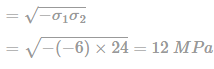*Answer can only contain numeric values
Practice Test: Civil Engineering (CE)- 11 - Question 62

The figure shows reducing area conduit carrying water. The pressure p and velocity V are uniform across sections 1 and The density of water is 1000 kg/m3. If the total loss of head due to friction is just equal to the loss of potential head between the inlet and the outlet, then V2 in m/s will be _____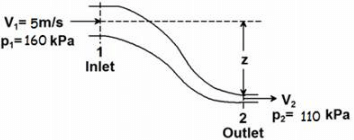Detailed Solution for Practice Test: Civil Engineering (CE)- 11 - Question 62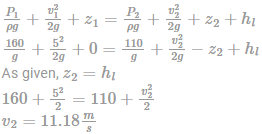*Answer can only contain numeric values
Practice Test: Civil Engineering (CE)- 11 - Question 63

A job having a processing time of 11 days is to be processed in scheduling operation. The job is to be delivered on 18th july 2018 but the job is received on 7th july. Find the critical ratio of the job to be processed.

Detailed Solution for Practice Test: Civil Engineering (CE)- 11 - Question 63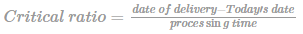= 18-7/11

= 11/11

= 1

Practice Test: Civil Engineering (CE)- 11 - Question 64

A rectangular beam of cross-section B × D has moment of resistance equal to M. A smaller square section is removed from the centre such that the moment of resistance of the remaining area is equal to half the initial MOR. The length of side of section removed is

Detailed Solution for Practice Test: Civil Engineering (CE)- 11 - Question 64

For solid section

Moment of resistance of the solid section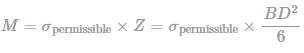For Hollow Section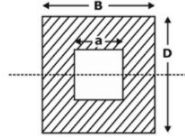Moment of inertia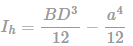Section modulus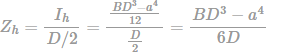Moment of Resistance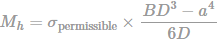Given

Mh = M/2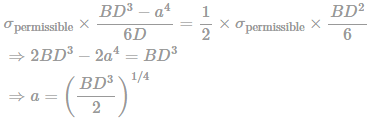Practice Test: Civil Engineering (CE)- 11 - Question 65

During the construction of a highway bridge, the average permanent load on the clay layer is expected to increase by about 95 kN/m3. The average effective overburden pressure at the middle of the clay layer is 190 kN/m3. Here, H is 4.5 m, Compression index is 0.26, e0 = 0.78, and coefficient of consolidation is 0.23 m2/month. The clay is normally consolidated. Determine the total primary consolidation settlement (in mm) and the ratio of pre-compression pressure to the effective stress of the bridge.

Detailed Solution for Practice Test: Civil Engineering (CE)- 11 - Question 65

The total primary consolidation settlement will be calculated as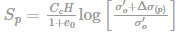On substituting the values in above equation Sp = 0.115m = 115mm

Ratio of pre-compression pressure to the effective stress is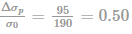## GATE Civil Engineering (CE) 2023 Mock Test Series

27 docs|296 tests
 Use Code STAYHOME200 and get INR 200 additional OFF Use Coupon Code
Information about Practice Test: Civil Engineering (CE)- 11 Page
In this test you can find the Exam questions for Practice Test: Civil Engineering (CE)- 11 solved & explained in the simplest way possible. Besides giving Questions and answers for Practice Test: Civil Engineering (CE)- 11, EduRev gives you an ample number of Online tests for practice

## GATE Civil Engineering (CE) 2023 Mock Test Series

27 docs|296 tests